Definitions of Square Dance Calls and Concepts

Index -->  Plus  |  A1  |  A2  |  C1  |  C2  |  C3A  |  C3B  |  C4  |  NOL  |
Definitions (Text Only) -->  Plus  |  A1  |  A2  |  C1  |  C2  |  C3A  |  C3B  |  C4  |  NOL  |
 Find call:

Fan CONCEPT \$B\$O(B \$B;O\$a\$,(B Arm Turn 1/2 (Swing) \$B\$G(B, \$B

Fan CONCEPT \$B\$,;H\$o\$l\$kBeI=E*\$JNc(B:

\$BCm
• Fan CONCEPT \$B\$O(B 'Spin The Top' \$B\$H(B 'Fan The Top' \$B\$N0c\$\$\$r0lHL2=\$7\$?\$b\$N\$G\$9(B.
• 'Spin' \$B\$G;O\$^\$k%3!<%k\$G\$O(B, 'Spin' \$B\$r(B 'Fan' \$B\$KJQ\$(\$^\$9(B (\$BNc\$(\$P(B, Spin Chain the Gears \$B\$O(B Fan Chain the Gears \$B\$H\$J\$j\$^\$9(B). \$BB>\$N%3!<%k\$G\$O(B 'Fan' \$B\$r%3!<%k\$NA0\$KF~\$l\$^\$9(B (\$BNc\$(\$P(B, Alter the Wave \$B\$O(B Fan Alter the Wave \$B\$H\$J\$j\$^\$9(B).
• \$B>e5-\$K<(\$7\$?%3!<%k\$O(B, \$B8~\$+\$\$9g\$C\$?(B Setup (Eight Chain Thru \$B\$^\$?\$O8~\$+\$\$9g\$C\$?(B Line) \$B\$+\$i\$b9T\$(\$^\$9(B. \$B\$=\$N>l9g\$O(B, Step To A Wave \$B\$r\$7\$F\$+\$i%3!<%k\$r9T\$\$\$^\$9(B.
• \$B%3!<%i!<\$O(B Fan any call \$B\$NBe\$o\$j\$K(B, Finish any call \$B\$H\$b%3!<%k\$G\$-\$^\$9(B.
• Although at first glance, you may think that Fan can always be replaced by Finish, this is not so. The "facing couples / ocean wave" rule applies to the Fan concept but not the Finish concept. For example, from an Eight Chain Thru formation, Fan Relay The Top is legal, whereas Finish Relay The Top is not.

•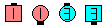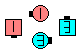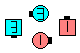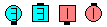Fan Alter The Wave\$B\$NA0(B Centers Arm Turn 3/4 as Ends U-Turn Back\$B\$N8e(B Counter Rotate 1/2\$B\$N8e(B Flip The Diamond\$B\$N8e(B (\$B=*\$o\$j(B)
Fan Chain Thru: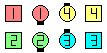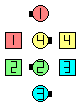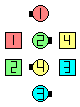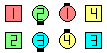Fan Chain Thru\$B\$NA0(B Centers Cast Off 3/4\$B\$N8e(B Very Centers Trade\$B\$N8e(B Centers Cast Off 3/4\$B\$N8e(B (\$B=*\$o\$j(B) (\$B\$H\$-\$K\$O(B Ends \$B\$K\$H\$C\$F(B, \$B\$H\$F\$bFq\$7\$/\$J\$j\$^\$9(B!)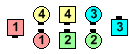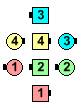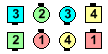Fan Relay The Shadow\$B\$NA0(B Center 6 Step To A Wave and Arm Turn 3/4as Very Ends Counter Rotate 1/4\$B\$N8e(B Centers Hinge and spread apartas Others do the Centers Part of Cast A Shadow\$B\$N8e(B (\$B=*\$o\$j(B)
\$BFinish CONCEPT.Choreography for Fan ConceptComments? Questions? Suggestions?

https://www.ceder.net/def/fan.php?level=C3B&language=japan
10-June-2023 14:28:45